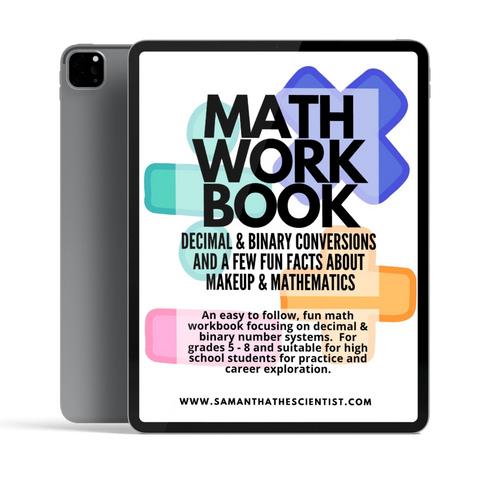# Decimal & Binary Math Ebook

## Decimal & Binary Math Ebook

Regular price \$12.00 USD
Regular price Sale price \$12.00 USD
Sale Sold out

# MATH WORKBOOK FOR TEENS## An easy-to-follow Math Workbook designed to help teens build the confidence and skills they need to excel in mathematics.

Whether they're in middle school or high school, this workbook can provide the foundation needed to understand complex equations.

This Decimal & Binary Math Ebook is an ideal math workbook for young teens.

It includes easy-to-follow exercises for decimal and binary conversions from 0 to 31.

With straightforward instructions and helpful practice examples, this Ebook can help learners master decimal and binary math.

## DECIMAL & BINARY MATH EBOOK INCLUDES:

• Easy to follow guidelines for Decimal & Binary Conversions from 0 to 31
• 15 Decimal Weight math questions
• 16 Decimal to Binary Conversions practice questions
• 28 Binary to Decimal Conversions practice questions
• 7 Decimal & Binary number chart practice questions
• Multiplication practice pages
• Makeup Lab Coloring Book## Why are decimal and binary numbers important for teens to learn?

This Math Workbook explores decimal and binary numbers from 0 to 31.  Understanding decimal and binary numbers is crucial for teens in today's digital world. These number systems are the foundation of computer programming and data representation. By learning about decimal and binary numbers, teens can develop essential skills that will benefit them in various fields, including computer science, engineering, and mathematics.

## What are decimal and binary numbers?

Decimal numbers are the numbers we use in our everyday lives. They are based on the decimal system, which uses ten digits (0-9) to represent numbers. For example, the number 25 in decimal is written as 25.

On the other hand, binary numbers are the building blocks of digital technology. They use only two digits (0 and 1) to represent numbers. In binary, the number 25 is written as 11001.

## How do teens benefit from learning about decimal and binary numbers?

1. Enhanced problem-solving skills: Learning to convert between decimal and binary numbers requires logical thinking and problem-solving abilities. These skills are transferable to various real-life situations and can help teens approach complex problems with a structured mindset.

2. Improved computational thinking: Decimal and binary numbers are the basis of computer programming. By understanding these number systems, teens can develop computational thinking skills, which involve breaking down problems into smaller steps and analyzing patterns.

3. Career opportunities: In today's technology-driven world, knowledge of decimal and binary numbers opens up numerous career opportunities. Fields such as computer science, software engineering, data analysis, and cybersecurity require a solid understanding of these number systems.

Learning about decimal and binary numbers is not only important for teens interested in pursuing careers in technology but also for their overall cognitive development. These number systems provide a solid foundation for problem-solving, computational thinking, and logical reasoning. By mastering decimal and binary conversions, teens can unlock a world of opportunities and gain a deeper understanding of the digital world around them.